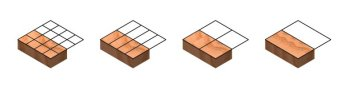Computer-Aided Design Engineering Programming Architectural Design Mechanical Design Civil Design Energy Management Electrical Design

Network
Engineer

Project
Management
 Table of ContentsWhen working with fractions, we need to be able to convert the value to a proportional number so we can add or subtract them. As shown in the image above, by dividing both the numerator and the denominator by two reduces 8/16 to 4/8, then 4/8 to 2/4, and finally 2/4 to 1/2. Therefore, we now know that 8/16 = 4/8 = 2/4 = 1/2. We could have easily gone in the other direction, multiplying both the numerator and the denominator by two never completing the task since both parts of the fraction would go to infinity. At World Class CAD, we use computer applications to solve every type of challenge. Working with fractions is just one. Interested in food density and yield charts, check out these links: Approximate Yields of Fruits and Vegetables from Restaurantcoachingsolutions.com Culinary Math Web Page Fibonacci Sequences Sequences Phi, Fibonacci Spiral and the Golden Ratio Powers of Phi and Determining a Fibonacci Number Similarity and Gnomons Quiz 1 Test 1 Test 1b Test 1d Percentages Purchasing Power 1 Purchasing Power 2 Basic Percentages Percent of Error and More Compund Interest Compound Interest Graph Paper Practice Problems Quiz 2 Test 2 Test 2b Population Population Growth Population Growth 2 Population Growth 3 Population Growth 4 Population Growth Review Quiz 3 Test 3 Test 3b Graphing Bar Charts Mean Median Mode and Bar Charts Pie Graph - Graphics Pie Charts Pie Chart Blank Bar and Pie Chart Review Quiz 3 Review Test 4 Test 4b Probability Flip a Coin, Roll a Die and Draw a Card Sample Spaces Combinations More Combinations Permutations and Combinations Quiz 4 Test 5 Test 5b Test 5c Test 5d Bell Curves, Standard Deviation, Weighted Averages Bell Curve Lab Chart 1 Chart 2 and 3 Weighted Averages Quiz 5 Quiz 5-1 Quiz 5-1b Review Test 6 Test 6b Test 6c Election Math Election by Elimination and Borda Count Method Banzhaf Power Index Shapley Shubik Power Index Review Quiz 6 Sharing and Apportionment Sharing Fair Divisions Apportionment Review Quiz 7 Test 7 Venn Diagrams Venn Diagram1 Venn Diagram2 Quiz 8